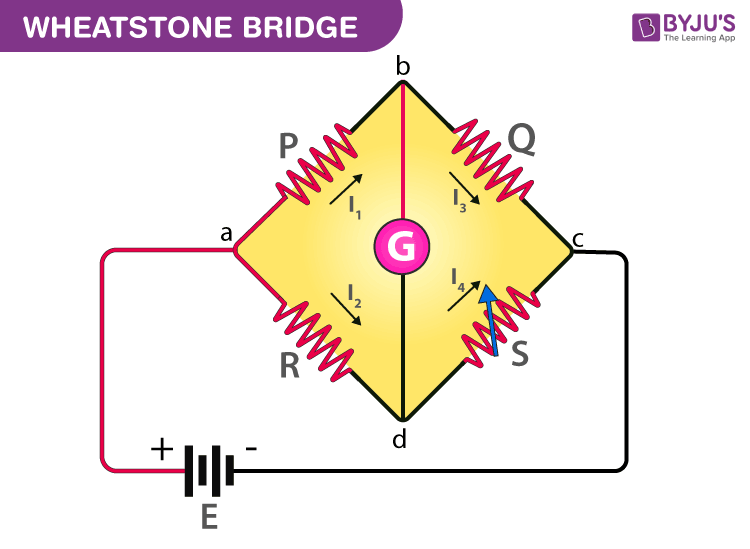How does a bridge circuit work?

The standard bridge circuit is called a Wheatstone bridge.Wheatstone bridge is used to determine the unknown resistance by balancing two legs of the bridge circuit, of which one leg includes the component of unknown resistance.

The Wheatstone bridge works on the principle of null deflection, where the ratio of resistances are equal and no current flows through it. Under normal conditions, the bridge is unbalanced and the current flows through the galvanometer. In a balanced condition, no current flows through the galvanometer and this condition can be achieved by adjusting the values of the known resistance and variable resistance.

The following condition exists when the current through a galvanometer is zero in the above circuit.

I1P=I2R

For the measurement of unknown resistance, the bridge circuit consists of a resistor whose value is unknown and three resistors of known value. Either of the three known resistors can be adjusted and replaced until the bridge is balanced. When the balance has been reached, the resistance of the unknown resistor can be determined from the ratios of the known resistances.(1)(0)